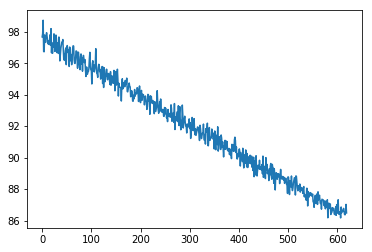# One-hot encoding

Hi.

I’m newbie at Machine learning and PyTorch.
I want to know how to work one-hot encoding, so wrote extremely simple example.

``````import torch
from torch import nn, optim

# Only 2 category
X_train = []
y_train = []
for i in range(1000):
if i % 2 == 0 :
X_train.append([1,0])
y_train.append(-10.0)
else :
X_train.append([0,1])
y_train.append(+10.0)

X_train = torch.Tensor(X_train)
y_train = torch.Tensor(y_train)

#
ds_train = TensorDataset(X_train, y_train)

# network
net = nn.Sequential(nn.Linear(2,1))

#
loss_fn = nn.L1Loss()

# loop
losses = []
net.train()
lastTime = time.time()
for epoc in range(10):
y_pred = net(data)
loss = loss_fn(target,y_pred)
loss.backward()
optimizer.step()
losses.append(loss.data)

%matplotlib inline
from matplotlib import pyplot as plt
plt.plot(losses[:])
``````

But this optimizer predict noting, always outputs near zero.
What’s wrong with this?

Thanks

L1 loss is not a proper loss function for one-hot elements. You should try cross-entropy loss (assuming that there is no bug)

Hello, I don’t think `nn.CrossEntropyLoss` is actually helpful here. I would recommend using `nn.MSELoss`. You can find some discussion about the differences between the two here:

Therefore, after some minor changes to your code, I managed to get it to converge:

``````import torch
from torch import nn, optim
import time  # Added the module

# Only 2 category
X_train = []
y_train = []
for i in range(1000):
if i % 2 == 0 :
X_train.append([1,0])
y_train.append([-10.0])  # Change from (-10.0) to ([-10.0])
else :
X_train.append([0,1])
y_train.append([+10.0])  # Change from (+10.0) to ([+10.0])

X_train = torch.Tensor(X_train)
y_train = torch.Tensor(y_train)

#
ds_train = TensorDataset(X_train, y_train)

# network
net = nn.Sequential(nn.Linear(2,1))

#
loss_fn = nn.MSELoss()  # Change from L1Loss to MSELoss

# loop
losses = []
net.train()
lastTime = time.time()
for epoc in range(10):
y_pred = net(data)
loss = loss_fn(y_pred, target)  # Change (target, y_pred) to (y_pred, target)
loss.backward()
optimizer.step()
losses.append(loss.data)

%matplotlib inline
from matplotlib import pyplot as plt
plt.plot(losses[:])
``````

And this is the loss I am getting for 10 epochs:However, it seems to converge a bit before 200 epochs: